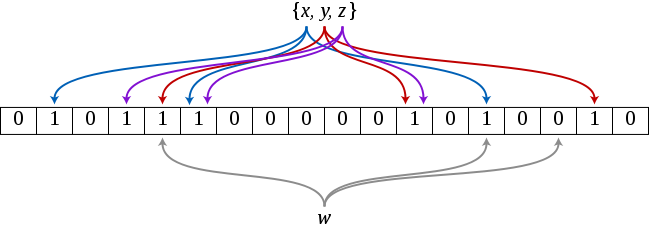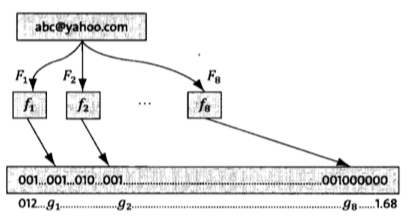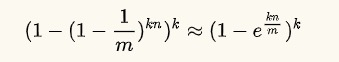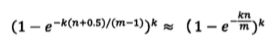### 使用场景

1. 有的黑客为了让服务宕机，他们会构建大量不存在于缓存中的key向服务器发起请求，在数据量足够大的情况下，频繁的数据库查询可能导致DB挂掉。布隆过滤器很好的解决了缓存击穿的问题。
2. 反垃圾邮件，从数十亿个垃圾邮件列表中判断某个邮箱是否是垃圾邮箱。
3. 网页爬虫对URL去重，防治爬取相同的URL地址
4. ...

### 算法描述### 举例说明### 误判概率的证明和计算### PHP实现

```class BloomFilterHash
{
/**
* 由Justin Sobel 编写的按位散列函数.
*
* @param string \$string
* @param null \$len
* @return int
*/
public function JSHash(\$string, \$len = null)
{
\$hash = 1315423911;
\$len || \$len = strlen(\$string);
for (\$i = 0; \$i < \$len; \$i ++) {
\$hash ^= ((\$hash << 5) + ord(\$string[\$i]) + (\$hash >> 2));
}

return (\$hash % 0xFFFFFFFF) & 0xFFFFFFFF;
}

/**
* 该哈希算法基于AT＆T贝尔实验室的Peter J. Weinberger的工作。
* Aho Sethi和Ulman编写的“编译器（原理，技术和工具）”一书建议使用采用此特定算法中的散列方法的散列函数。
*
* @param string \$string
* @param null \$len
* @return int
*/
public function PJWHash(\$string, \$len = null)
{
\$bitsInUnsignedInt = 4 * 8;
\$threeQuarters = (\$bitsInUnsignedInt * 3) / 4;
\$oneEighth = \$bitsInUnsignedInt / 8;
\$highBits = 0xFFFFFFFF << (int) (\$bitsInUnsignedInt - \$oneEighth);
\$hash = 0;
\$test = 0;
\$len || \$len = strlen(\$string);
for (\$i = 0; \$i < \$len; \$i ++) {
\$hash = (\$hash << (int) (\$oneEighth)) + ord(\$string[\$i]);
}
\$test = \$hash & \$highBits;
if (\$test != 0) {
\$hash = ((\$hash ^ (\$test >> (int)(\$threeQuarters))) & (~\$highBits));
}
return (\$hash % 0xFFFFFFFF) & 0xFFFFFFFF;
}

/**
* 类似PJW Hash功能，但是针对32位处理器做了调整。它是基于unix系统上的widely使用哈希函数。
*
* @param string \$string
* @param null \$len
* @return int
*/
public function ELEHash(\$string, \$len = null)
{
\$hash = 0;
\$len || \$len = strlen(\$string);
for (\$i = 0; \$i < \$len; \$i++) {
\$hash = (\$hash << 4) + ord(\$string[\$i]);
\$x = \$hash & 0xF0000000;
if (\$x != 0) {
\$hash ^= (\$x >> 24);
}
\$hash &= ~\$x;
}

return (\$hash % 0xFFFFFFFF) & 0xFFFFFFFF;
}

/**
* 这个哈希函数来自Brian Kernighan和Dennis Ritchie的书“The C Programming Language”。
* 它是一个简单的哈希函数，使用一组奇怪的可能种子，它们都构成了31 .... 31 ... 31等模式，它似乎与DJB哈希函数非常相似。
*/
public function BKDRHash(\$string, \$len = null)
{
\$seed = 131;  # 31 131 1313 13131 131313 etc..
\$hash = 0;
\$len || \$len = strlen(\$string);
for (\$i=0; \$i<\$len; \$i++) {
\$hash = (int) ((\$hash * \$seed) + ord(\$string[\$i]));
}
return (\$hash % 0xFFFFFFFF) & 0xFFFFFFFF;
}

/**
* 这是在开源SDBM项目中使用的首选算法。
* 哈希函数似乎对许多不同的数据集具有良好的总体分布。它似乎适用于数据集中元素的MSB存在高差异的情况。
*/
public function SDBMHash(\$string, \$len = null)
{
\$hash = 0;
\$len || \$len = strlen(\$string);
for (\$i=0; \$i<\$len; \$i++) {
\$hash = (int) (ord(\$string[\$i]) + (\$hash << 6) + (\$hash << 16) - \$hash);
}
return (\$hash % 0xFFFFFFFF) & 0xFFFFFFFF;
}

/**
* 由Daniel J. Bernstein教授制作的算法，首先在usenet新闻组comp.lang.c上向世界展示。
* 它是有史以来发布的最有效的哈希函数之一。
*/
public function DJBHash(\$string, \$len = null)
{
\$hash = 5381;
\$len || \$len = strlen(\$string);
for (\$i=0; \$i<\$len; \$i++) {
\$hash = (int) ((\$hash << 5) + \$hash) + ord(\$string[\$i]);
}
return (\$hash % 0xFFFFFFFF) & 0xFFFFFFFF;
}

/**
* Donald E. Knuth在“计算机编程艺术第3卷”中提出的算法，主题是排序和搜索第6.4章。
*/
public function DEKHash(\$string, \$len = null)
{
\$len || \$len = strlen(\$string);
\$hash = \$len;
for (\$i=0; \$i<\$len; \$i++) {
\$hash = ((\$hash << 5) ^ (\$hash >> 27)) ^ ord(\$string[\$i]);
}
return (\$hash % 0xFFFFFFFF) & 0xFFFFFFFF;
}

/**
* 参考 http://www.isthe.com/chongo/tech/comp/fnv/
*/
public function FNVHash(\$string, \$len = null)
{
\$prime = 16777619; //32位的prime 2^24 + 2^8 + 0x93 = 16777619
\$hash = 2166136261; //32位的offset
\$len || \$len = strlen(\$string);
for (\$i=0; \$i```
```abstract class BloomFilterRedis
{
/**
* 需要使用一个方法来定义bucket名字.
*/
protected \$bucket;

protected \$hashFunction;

public function __construct()
{
if (!\$this->bucket || !\$this->hashFunction) {
throw new Exception("需要定义bucket和hashFunction");
}

\$this->Hash = new BloomFilterHash;
\$this->Redis = new \Redis();   // 假设已经连接好了
\$this->Redis->connect('127.0.0.1');
}

/**
* @param \$string
* @return array
*/
public function add(\$string)
{
\$pipe = \$this->Redis->multi();
foreach (\$this->hashFunction as \$function) {
\$hash = \$this->Hash->\$function(\$string);
\$pipe->setBit(\$this->bucket, \$hash, 1);
}
return \$pipe->exec();
}

/**
* 查询是否存在，不存在的一定不存在，存在的可能存在误判.
*
* @param \$string
* @return bool
*/
public function exists(\$string)
{
\$pipe = \$this->Redis->multi();
\$len = strlen(\$string);
foreach (\$this->hashFunction as \$function) {
\$hash = \$this->Hash->\$function(\$string, \$len);
\$pipe = \$pipe->getBit(\$this->bucket, \$hash);
}
\$res = \$pipe->exec();
foreach (\$res as \$bit) {
if (\$bit == 0) {
return false;
}
}

return true;
}
}
```
```class FilteRepeatedComments extends BloomFilterRedis
{
protected \$bucket = 'rptc';

protected \$hashFunction = array('BKDRHash', 'SDBMHash', 'JSHash');
}
```